IEEE754 floating-point representation

Number representation

Floating point system sign bit exponent bit fraction bit(mantissa)
32-bits 1 8 23
64-bits 1 11 52

Following are representations ofin different floating point system. (s stands for sign, e for exponent, f for fraction)

32bits:

seeeeeeeefffffffffffffffffffffff
00111111100000000000000000000000

64bits:

seeeeeeeeeeeffffffffffffffffffffffffffffffffffffffffffffffffffff
0011111111110000000000000000000000000000000000000000000000000000

Calculating floating point value

As we can image, the way to calculate the a floating point value is simply like:where for sign bit: 0 = positive number, 1 = negative number

But there are a few things to notice:

First: In order to introduce small numbers like 0.0000123 into floating point system, we have to allow exponent to be negative. Therefore, the exponent part has bias, which equals to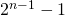where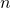is number of exponent bits in the floating point system, and the actual exponent representation equals towhere the bias in 32-bit system is 127, and that in 64-bit system is 1023.

Therefore, exponent 0 in 32-bit system is like:

01111111

Second: A nice little optimization is now available to us in base two, since binary has only one possible non-zero digit: 1. Thus, we can just assume a leading digit of 1, and don’t need to store it in the floating-point representation. As a result, we can assume a leading digit of 1 without storing it, so that a 32-bit floating-point value effectively has 24 bits of mantissa: 23 explicit fraction bits plus one implicit leading bit of 1.1

As the result, the real value of fraction part equals to: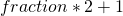because we have an “invisible”at the end of the mantissa.

Therefore, fraction 1 in 32-bit system is just an all-zero string.

00000000000000000000000

So to conclude, we can generalize the following equation to calculate the real value of of a floating-point number:
1. for 32-bit float:2. for 64-bit float: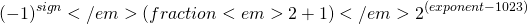Exceptions

Zero

Condition: When exponent part = 0 and fraction part = 0.

Since mantissa part always assumes an “1” at the end of fraction part, we defaults zero to be a number with exponent part = 0 and fraction part = 0.

Additionally, due to the existence of sign bit, there exists two zeros in floating number: +0 and -0, which are represented differently in bit level.

Infinity

Condition: When exponent part =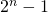and fraction part = 0.

Not A Number

Condition: When exponent part =and fraction part is not 0

Special Operations

Operation Result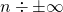0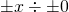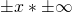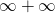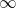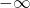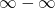NaN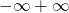NaN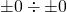NaNNaN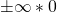NaN

1. https://steve.hollasch.net/cgindex/coding/ieeefloat.html